# The greeks

Managing the risk of an option can be quite difficult especially due to its nonlinear payoff profile. Price or interest rate changes, time accrual, volatility jumps can both have a high and peculiar impact on the option value.

## Delta

The option delta is the first and most straightforward variable of the greeks. In mathematical terms it is the first derivative of the underlying price. It measures how much the option value is going to change when the underlying price changes. The delta ranges from -1 till 1. A delta of 1 means that the option price increases or decreases with the same amount as the underlying. A delta of -1 has the same but option and underlying value will move in the opposite direction. Call options (long) have a delta ranging from 0 till 1. Put options (long) hold deltas going from -1 till 0.Based on the Black-and-Scholes model, assuming no dividend yield, the delta of European call and European put options can be calculated as follows, in which N stands for the standard normal cumulative distribution.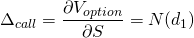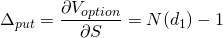## Gamma

The second greek is called the gamma. In mathematical terms in is the second derivative of the underlying price. Stated differently, gamma is the first derivative of the delta. It thus measures how much the delta of the option is changing when the underlying price changes. Also the gamma for european options can be derived from the Black-and-Scholes model. Note that the calculation of gamma is based on the standard normal distribution and not the cumulative one.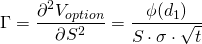## Theta

The third greek, theta, measures how much the option value changes when time goes by. The option theta is as a result negative for both calls and puts (long). As time pass, the option becomes worth less. The decay in price due to time is not linear. Especially near expiration, the option starts to lose a lot of its value in short time periods.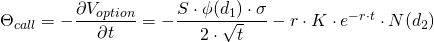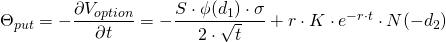## Rho

Rho measures how much the option value is changing when the risk free rate changes, directly. Meaning that the impact of the interest rate on the underlying price which is in turn affects the option value is left out.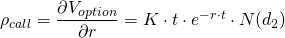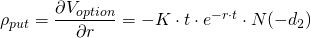## Vega

The final variable of the greeks is called vega. It measures how much the option value is going to change when the volatility of the underlying changes. An increase in volatility leads to an increase of value for both call and put options. Although sounding counterintuitive, both values increase due to the rising probability of ending up in the money. With high volatility either the underlying is more likely to increase or decrease a lot in price, more than remaining close to the same value.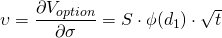## Summary

The option greeks are delta, gamma, theta, rho and vega. They allow managing the risk of an option or option portfolio with respect to price changes, time accrual, interest rates and volatility.